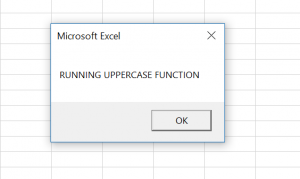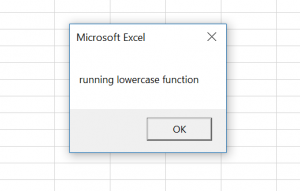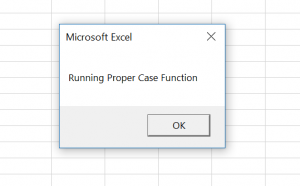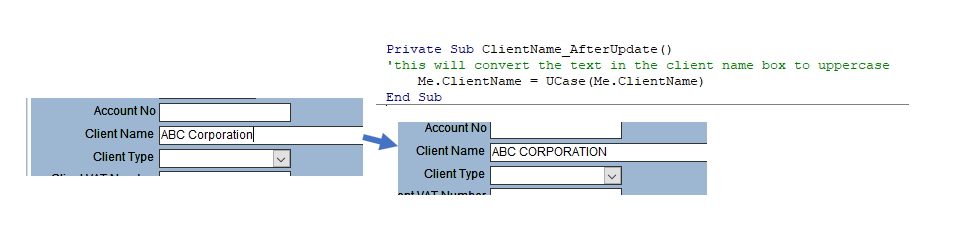# VBA Upper, Lower, and Proper Case – Case Functions

This tutorial will demonstrate how to use the UCASE, LCASE and STRCONV functions in VBA.

While working in VBA, you often need to convert strings into lowercase, uppercase or proper case. This is possible by using the UCase, LCase and StrConv functions.

These functions are important when manipulating strings in VBA, as VBA is case sensitive. If you wish to make VBA case-insensitive, you need to add Option Compare Text at the top of your module. You can find out more about this here: Prevent VBA Case Sensitive

## UCase – Convert String to Upper Case

The UCase function in VBA converts all letters of a string into uppercase. There is only one argument, which can be a string, variable with string or a cell value. This function is often used if you want to compare two strings. Here is the code for the UCase function:

``````Dim strText As String
Dim strTextUCase As String

strText = "running Uppercase function"

strTextUCase = UCase(strText)

MsgBox strTextUCase``````

In the example, we want to convert all letters of the strText variable to upper case and assign the converted string to the strTextUCase variable. At the end we call the message box with the converted string:## LCase – Convert String to Lower Case

If you want to convert all letters of a string into lower cases, you need to use the LCase function. This function has one argument, the same as the UCase. This is the code for the LCase function:

``````Dim strText As String
Dim strTextLCase As String

strText = "RUNNING lowerCASE FUNCTION"

strTextLCase = LCase(strText)

MsgBox strTextLCase``````

In this example, we convert all letters of the string variable strText into lower case. After that, the converted string is assigned to the variable strTextLCase.## StrConv – Convert String to Proper Case

The StrConv function enables you to convert a string of text into proper case. The function has two arguments. First is the string that you want to convert. The second is the type of the conversion which you want. In order to convert a string to a proper case, you need to set it to vbProperCase. The code for the function is:

``````Dim strText As String
Dim strTextProperCase As String

strText = "running proper case function"

strTextProperCase = StrConv(strText, vbProperCase)

MsgBox strTextProperCase``````

You will see on the example how the function works. It takes the string from the cell B1, converts it to proper case and returns the value in the cell A1.## StrConv – Convert String to Upper or Lower Case

Using the StrConv function, you can also convert a string to upper or lower cases. To do this, you just need to set the second argument to the vbUpperCase or vbLowerCase:

``strTextConverted = StrConv(strText, vbUpperCase)``
``strTextConverted = StrConv(strText, vbLowerCase)``

## VBA Upper, Lower, and Proper Case – Case Functions in Access

All of the above examples work exactly the same in Access VBA as in Excel VBA.

``````Private Sub ClientName_AfterUpdate()
'this will convert the text in the client name box to uppercase
Me.ClientName = UCase(Me.ClientName)
End Sub``````Stop searching for VBA code online. Learn more about AutoMacro - A VBA Code Builder that allows beginners to code procedures from scratch with minimal coding knowledge and with many time-saving features for all users!